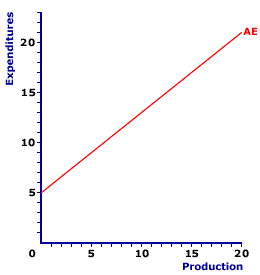Sunday  October 24, 2021
 AmosWEB means Economics with a Touch of Whimsy!POLLUTION: Any waste that imposes an opportunity cost when it's returned to the natural environment. Pollution is one of the more prevalent examples of an externality cost and market failure. Examples include, but by no means are limited to, car exhaust, municipal sewage, industrial waste, and agricultural chemical runoff from farms. Pollution waste can be classified as degradable, persistent, or nondegradable, depending on how easily it can be broken down into nonharmful form by the natural environment. Pollution problems can never be eliminated, but they can be handled with efficiency if the amount of pollution is such that the cost of damages is the same as the cost of cleanup.SLOPE, AGGREGATE EXPENDITURES LINE:

The positive slope of the aggregate expenditures line is the sum of the marginal propensity to consume (MPC), marginal propensity to invest (MPI), and marginal propensity for government purchases (MPG), less the marginal propensity to import (MPM). This slope is greater than zero but less than one, reflecting induced expenditures by the four macroeconomic sectors (household, business, government, and foreign). The slope of the aggregate expenditures line determines the magnitude of the multiplier process.
Aggregate Expenditures LineThe aggregate expenditures line, which embodies the key Keynesian principle of effective demand, shows the relation between aggregate expenditures and the actual level of aggregate income or production in the domestic economy. The income and production measures commonly used are national income and gross domestic product.

The two basic types of expenditures--induced and autonomous--are indicated by the aggregate expenditures line.

A representative aggregate expenditures line is presented in the exhibit to the right. This red line, labeled AE in the exhibit, is positively sloped, indicating that greater levels of income generate greater aggregate expenditures by the four sector. This positive relation is primarily based on the Keynesian psychological law indicating that consumption expenditures are induced by household income. However, this positive slope is reinforced and augmented by induce investment, government purchases, and net exports.

The slope of the aggregate expenditures line presented here is positive, but less than one. Click the [Slope] button to illustrate. In fact, the slope of the aggregate expenditures line is numerically based on the marginal expenditure propensities for any of the induced expenditures included in the aggregate expenditures line. For a "complete" four-sector aggregate expenditures line, the slope is equal to the sum of the marginal propensity to consume (MPC), marginal propensity to invest (MPI), and marginal propensity for government purchases (MPG), less the marginal propensity to import (MPM). If induced taxes are also included, then the slope is adjusted for the marginal tax rate.

To illustrate the connection between slope and the marginal expenditure propensities, consider the equation for the slope of the aggregate expenditures line, specified as the "rise" over the "run." The rise is the change in aggregate expenditures measured on the vertical axis and the run is the change in income (or production) measured on the horizontal axis.

 slope = riserun = change in aggregate expenditureschange in income
However, for any induced movement on the aggregate expenditures line, the change in aggregate expenditures includes changes in consumption expenditures, investment expenditures, government purchases, or net exports. The slope of the aggregate expenditures line can thus be specified as based on each of these expenditures.
 slope = change in (consumption + investment + government purchases + net exports)change in income
This can be rewritten as:
 slope = change inconsumptionchange inincome + change ininvestmentchange inincome + change ingovernment purchaseschange inincome + change innet exportschange inincome
Because the induced change in net exports is attributable totally to the induced change in imports and because imports are subtracted for exports to derive net exports, the change in net exports is equal to the negative change in imports. The slope can be rewritten as:
 slope = change inconsumptionchange inincome + change ininvestmentchange inincome + change ingovernment purchaseschange inincome - change inimportschange inincome
Each of these terms is a corresponding marginal propensity.
 slope = marginal propensityto consume + marginal propensityto invest + marginal propensityfor government purchases - marginal propensityto import
Or, using standard abbreviations that are both symbolic and a little easier to remember.
 slope = MPC + MPI + MPG - MPM
Because each of these marginals is the slope of the corresponding expenditure line (consumption line, investment line, government purchases line, and net exports line), the slope of the aggregate expenditures line is the summation of the slopes of the lines for each of the four aggregate expenditures.

The positive slope of the aggregate expenditures line reflects induced expenditures, which are aggregate expenditures that depend on the level of income or production. If the aggregate economy has more income, then the four macroeconomic sectors are induced to undertake additional expenditures. Of course, a drop in aggregate income or production induces the sectors to reduce expenditures.

 <= SLOPE, AGGREGATE DEMAND CURVE SLOPE, CONSUMPTION LINE =>Recommended Citation:

SLOPE, AGGREGATE EXPENDITURES LINE, AmosWEB Encyclonomic WEB*pedia, http://www.AmosWEB.com, AmosWEB LLC, 2000-2021. [Accessed: October 24, 2021].

Check Out These Related Terms...

Or For A Little Background...

And For Further Study...
Search Again?PURPLE SMARPHIN[What's This?] Today, you are likely to spend a great deal of time at an auction wanting to buy either 500 feet of coaxial cable or a coffee cup commemorating the 1960 Presidential election. Be on the lookout for small children selling products door-to-door.Your Complete ScopeA thousand years before metal coins were developed, clay tablet "checks" were used as money by the Babylonians."Do not let what you cannot do interfere with what you can do. "-- John Wooden, basketball coachAEAAmerican Economic AssociationA PEDestrian's Guide Xtra CreditTell us what you think about AmosWEB. Like what you see? Have suggestions for improvements? Let us know. Click the User Feedback link.| | | | | | | | | | |
| | | |

Thanks for visiting AmosWEB